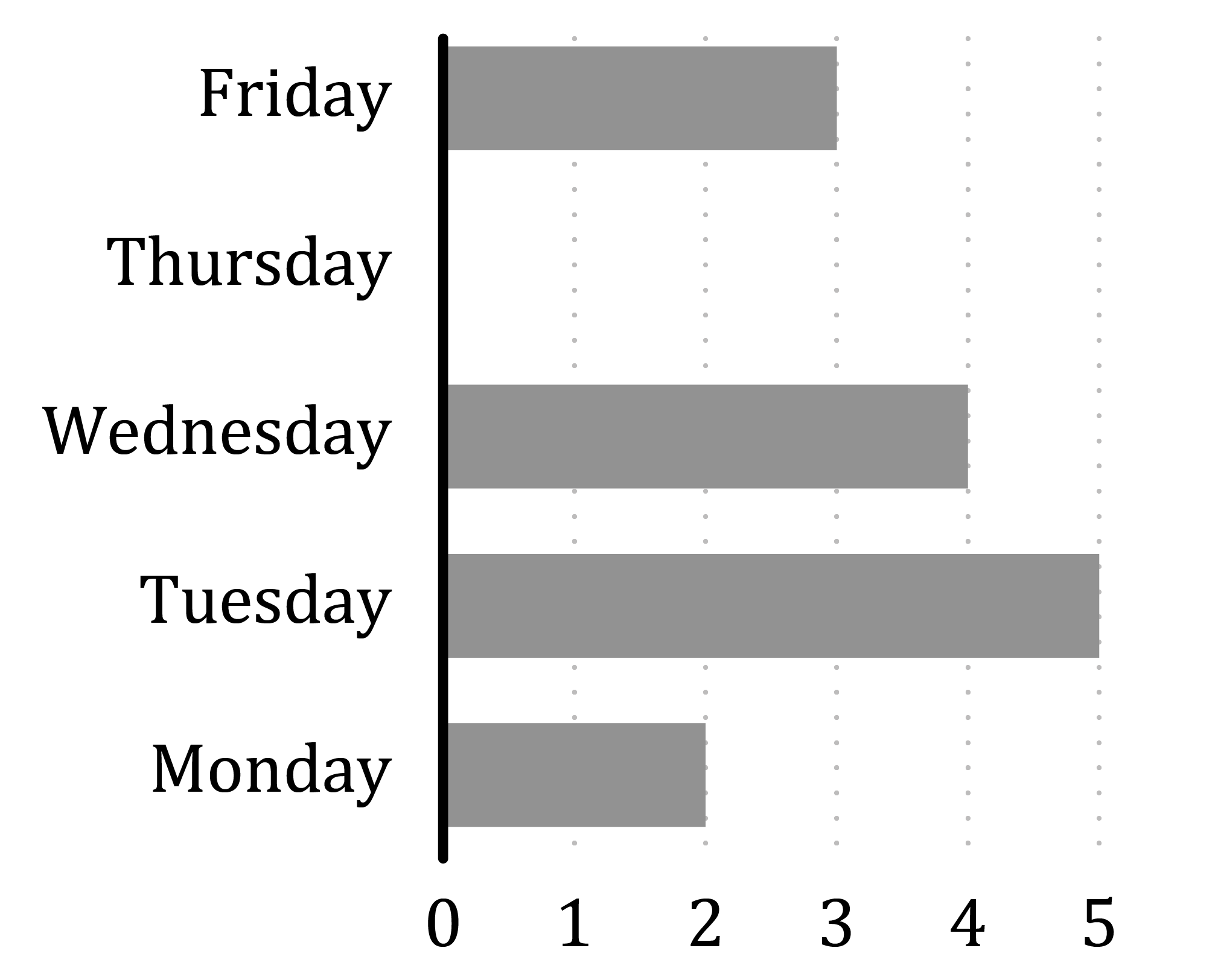Chapter overviewMaths

Number and place value

Multiplication and division

Fractions

Measurement

Geometry - properties of shapes

Statistics

Maths

# Bar charts0%

Summary

# Bar Charts

## In a nutshell

Bar charts help you to visualise data at a glance. They make information involving 'how many' easy to compare and understand.

### Definitions

#### Frequency

How many of something.

#### scale

The amount that the frequency axis goes up in.

## Interpreting bar charts

A bar chart has two labelled axes: one for frequency and one for categories. The height of each bar represents the frequency of items in that category.

##### Example 1

Bar chart to show students' favourite animals

Note: The scale of a bar chart could be anything from ones to twos to hundreds to thousands!

## Presenting data using bar charts

You can draw a bar chart from a table to present the data in a more visual way.

### Drawing bar charts

#### ​​procedure

 1 Draw and label the axes: one for frequency and one for the categories. 2 Pick an appropriate scale for the frequency. 3 Draw the bars so that their height represents the right frequency.
##### Example 2

Here the bar chart has been drawn from the tally chart shown below.

 ​$\begin{array}{c|c|c}\text{Day}&\text{Tally}&\text{Total} \\\hline\text{Monday} & || & 2 \\ \hline\text{Tuesday} & \cancel{||||} & 5 \\\hline\text{Wednesday} & |||| & 4 \\\hline\text{Thursday} & & 0 \\\hline\text{Friday} & ||| & 3 \end{array}$​​ ​ Bar chart to show carrots eaten by a rabbit on each day of the week.FAQs

• Question: How do I draw a bar chart?

Answer: 1. Draw and label the axes: one for frequency and one for the categories. 2. Pick an appropriate scale for the frequency. 3. Draw the bars so that their height represents the right frequency.

• Question: What are bar charts?

Answer: Bar charts help you to visualise data at a glance. They make information involving 'how many' easy to compare and understand.

Theory

Exercises